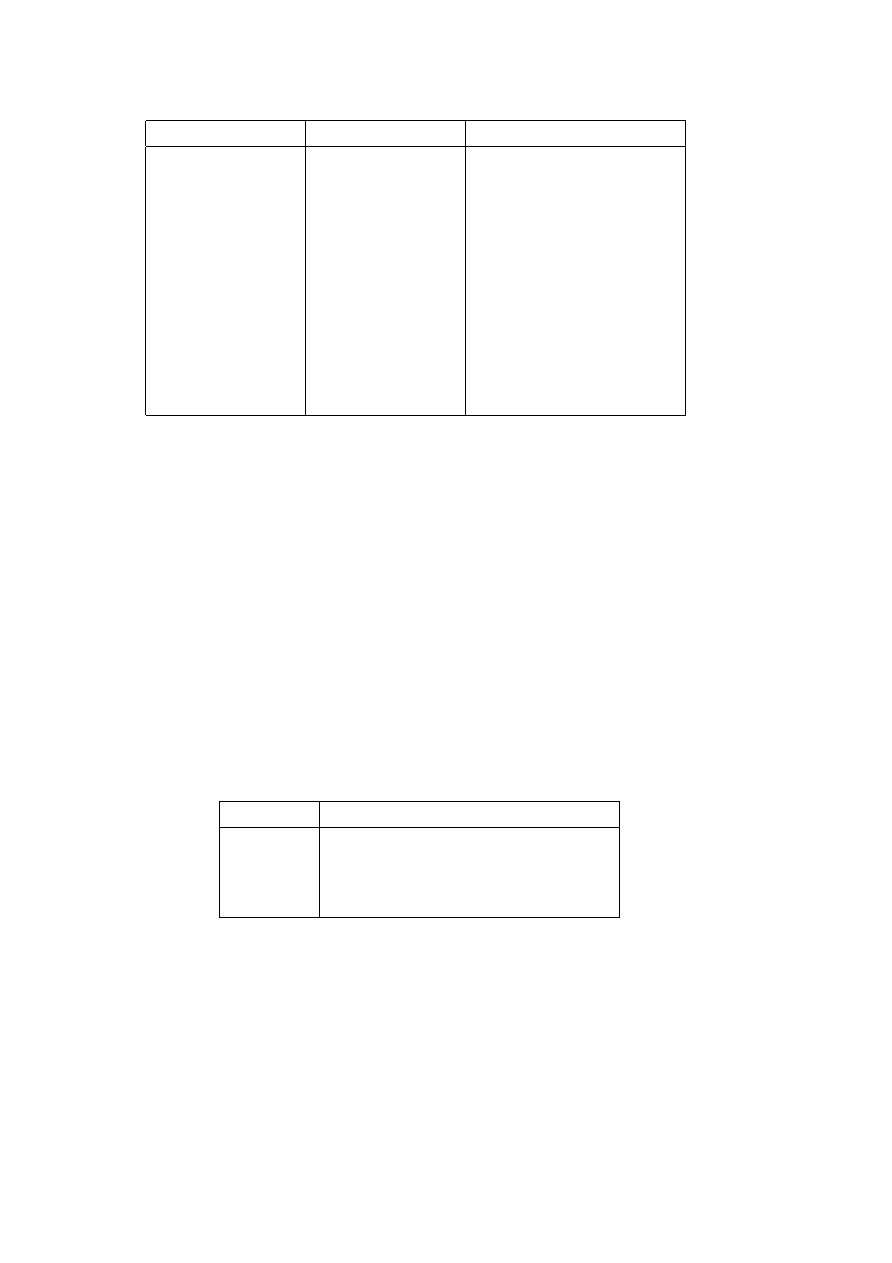ISO/IEC 10918-1 : 1993(E)
Table F.5 ­ Statistical model for AC coefficient coding
Context-index
Value
Coding decision
SE
3
×
(K ­ 1)
K
=
EOB
S0
SE
+
1
V
=
0
SS
Fixed estimate
Sign of V
SN,SP
S0
+
1
Sz
<
1
X1
S0
+
1
Sz
<
2
X2
AC_Context(K)
Sz
<
4
X3
X2
+
1
Sz
<
8
.
.
.
.
.
.
X15
X2
+
13
Sz
<
2
15
M2
X2
+
14
Magnitude bits if Sz
<
4
M3
X3
+
14
Magnitude bits if Sz
<
8
.
.
.
.
.
.
M15
X15
+
14
Magnitude bits if Sz
<
2
15
F.1.5
Extended sequential DCT-based Huffman encoding process for 12-bit sample precision
This process is identical to the sequential DCT process for 8-bit precision extended to four Huffman table destinations as
documented in F.1.3, with the following changes.
F.1.5.1
Structure of DC code table for 12-bit sample precision
The two's complement difference magnitudes are grouped into 16 categories, SSSS, and a Huffman code is created for
each of the 16 difference magnitude categories.
The Huffman table for DC coding (see Table F.1) is extended as shown in Table F.6.
Table F.6 ­ Difference magnitude categories for DC coding
SSSS
Difference values
12
­4 095..­2 048,2 048..4 095
13
­8 191..­4 096,4 096..8 191
14
­16 383..­8 192,8 192..16 383
15
­32 767..­16 384,16 384..32 767
F.1.5.2
Structure of AC code table for 12-bit sample precision
The general structure of the code table is extended as illustrated in Figure F.11. The Huffman table for AC coding is
extended as shown in Table F.7.
102
CCITT Rec. T.81 (1992 E)